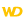Home |Sign in | EnglishHelpWLanguageWLanguage syntaxOperatorsBinary operatorsUseBinary operatorsShift operatorsOperator for direct access to the bitsSee alsoArithmetic operatorsAssignment operatorsBinary operatorsComparison operatorsExchange operator (<=>)Indirection operatorsList of operatorsLogical operatorsMultiline stringsOperator on AddressOperators on character stringsOverviewVarious operators
• Use
• Binary operators
• Binary AND, OR and exclusive OR
• Binary NOT
• Shift operators
• Syntaxes
• Notes
WINDEVWEBDEVWINDEV MobileOthersBinary operators
Use
The operations on binary values are performed:
• by the WLanguage functions: BinaryAND, BinaryOR, BinaryNOT, BinaryXOR
• by specific operators: binary operators, operators for right or left offset, operator for accessing the bits.Versions 16 and laterThis feature is available for Windows Phone applications.New in version 16This feature is available for Windows Phone applications.This feature is available for Windows Phone applications.Versions 18 and laterThis feature is available in Windows Store apps mode.New in version 18This feature is available in Windows Store apps mode.This feature is available in Windows Store apps mode.Versions 21 and laterThis feature is available in Universal Windows 10 App mode.New in version 21This feature is available in Universal Windows 10 App mode.This feature is available in Universal Windows 10 App mode.
Binary operators

Binary AND, OR and exclusive OR

The following syntaxes can be used:
• Binary AND: <Value 1> & <Value 2>
• Binary OR: <Value 1> | <Value 2>
• Binary exclusive OR: <Value 1> || <Value 2>
The type of result depends on the type of the operands:
 Value 2Value 1 Integer on 4 Integer on 8 Other Integer on 4 Integer on 4 Integer on 8 Integer on 4 Integer on 8 Integer on 8 Integer on 8 Integer on 8 Other Integer on 4 Integer on 8 Integer on 8

Binary NOT

The syntax is as follows: ~ <Value>
The type of result depends on the operand type:
 Operand Result Integer on 4 Integer on 4 Integer on 8 Integer on 8 Other Integer on 8
Shift operators

Syntaxes

• Offset to left:
<Value 1> bitLeftShift <Value 2>>
bitLeftShift(<Value 1>, <Value 2>)
• Offset to right:
<Value 1> bitRightShift <Value 2>>
bitRightShift(<Value 1>, <Value 2>)

Notes

• The bits of <Value 1> are shifted from <Value 2> bits to the right or to the left.
For example:
bitLeftShift(4,1) // Returns 8

Indeed, 4 in decimal corresponds to 0100 in binary. Shifted from 1 bit to the left, we get 1000 in binary that corresponds to 8 in decimal.
bitRightShift(4,2) // Returns 1

Indeed, 4 in decimal corresponds to 0100 in binary. Shifted from 2 bits to the right, we get 0001 in binary that corresponds to 1 in decimal.
• The bits that exceed the size of <Value 1> are ignored. For example:
bitLeftShift(4,30) // Returns 0
bitRightShift(4,4) // Returns 0
• If <Value 2> is greater than the size of <Value 1> (32 for a 4-byte integer and 64 for a 8-byte integer), the result is always equal to 0. For example:
bitLeftShift(4,35) // Returns 0
• The type of result depends on the type of the operand:
 Operand Value 1 Result Integer on 4 Integer on 4 Integer on 8 Integer on 8 Other Integer on 8The operator for left binary shift will reinject from the right the bits that exceed the operand size. The operator for right binary shift will reinject from the left the bits that exceed the operand size. For example:
e is int on 4
res is int on 4
e = 1
res = e bitLeftShift 32 // Returns 0 in WINDEV and 1 in JavaSyntax: <Value 1> [ <n> ]
This syntax is used to read or modify the value of <N> bit in the <Value 1> value.
Counting bits starts from 1 from left to right (from the lowest byte to the highest byte):
• 1 to 32 for an integer on 4 bytes,
• 1 to 64 for an integer on 8 bytes.
If the value of <n> is incorrect, the operation returns 0.
Examples:
// Positions the fifth and seventh bits to 1
n is int
n = 1
n = True
// Checks the value of bits 4, 5, 6 and 7
IF n THEN Trace(4) // not displayed
IF n THEN Trace(5) // displays 5
IF n THEN Trace(6) // not displayed
IF n THEN Trace(7) // displays 7
// Assign a value by modifying the bits
n is int = 0
// Switch on bit #1: n worth 1 in decimal
n = 1
// Switch on bit #8 as well: n worth 129 in decimal (2 power 0 + 2 power 7)
n = 1Syntaxes:
• <Value 1> [ <n>, wlInt_1 ]
• <Value 1> [ <n>, wlInt_2 ]
• <Value 1> [ <n>, wlInt_4 ]
These syntaxes are used to read or modify the value of integer on 1, 2 or 4 bytes in the <Value 1> value.
Possible values for <n> according to the type of <Value 1>:
 Value 1 wlInt_1 wlInt_2 wlInt_4 Integer on 4 1 to 4 1 to 2 1 Integer on 8 1 to 8 1 to 4 1 to 2

If the value of <n> is incorrect, the operation returns 0.Syntaxes:
• <Value 1> [ TO <n> ]
• <Value 1> [ <n> TO ]
• <Value 1> [ <n> TO <o> ]
• <Value 1> [ <n> ON <Number> ]
These syntaxes are used to read or modify the value corresponding to the specified bits.
Minimum version required
• Version 14